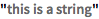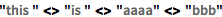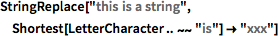Wolfram 语言：快速编程入门字符串观看视频

Wolfram 语言中的字符串用双引号指定（"..."）.

In:=1`"this is a string"`
Out=1In:=2`InputForm[%]`
Out=2<> 连接字符串：

In:=3`"this" <> "is" <> "aaaa" <> "bbb"`
Out=3Java 程序员备注：

<> 在 Wolfram 语言中特别用于连接字符串，目的是为避免使用 + 而产生模糊.

Python 程序员备注：

<> 在 Wolfram 语言中特别用于连接字符串，目的是为避免使用 + 而产生模糊.

Python 程序员备注：

Python 3 和 Wolfram 语言字符串都默认使用 Unicode. 在 Python 2 中，除非特别定义为 Unicode，字符串默认使用 ASCII.

In:=1`StringReplace["this is a string", Shortest[LetterCharacter ... ~~ "is"] -> "xxx"]`
Out=1RegularExpression 允许紧凑的正则表达式符号.

Java 程序员备注：

Python 程序员备注：

Wolfram 语言字符串模式用普通模式语法提供了强大的字符串匹配, 对于可读性和直观性进行了优化. Python 中最接近的是用 regex 的字符串匹配，其与 Wolfram 语言的 RegularExpression 函数作用相近.

In:=1`TemplateApply["number `` date: <* Now *>", {56}]`
Out=1Java 程序员备注：

TemplateApply 与 Java 的 System.out.printf 方法执行类似功能，但是 Wolfram 语言使用 `` 而不是 %无需类型指示语并可通过 <* ... *>允许行内计算.

Python 程序员备注：

TemplateApply 在 Wolfram 语言中与 Python 的 %format 函数的作用类似，除了 Python 中不支持用 <* ... *> 进行行内计算.